### Mathematical Description of NPSC

If a network programming problem with side constraints hasnodes,arcs,nonarc variables, andside constraints, then the formal statement of the problem solved by PROC NETFLOW is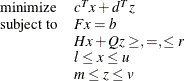where

•is the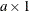arc variable objective function coefficient vector (the cost vector)

•is thearc variable value vector (the flow vector)

•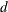is the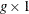nonarc variable objective function coefficient vector

•is thenonarc variable value vector

•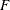is thenode-arc incidence matrix of the network, where

•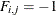if arcis directed from node•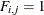if arcis directed toward node•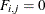otherwise

•is the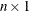node supply/demand vector, where

•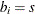if nodehas supply capability ofunits of flow

•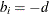if nodehas demand ofunits of flow

•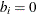if nodeis a trans-shipment node

•
is the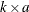side constraint coefficient matrix for arc variables, where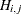is the coefficient of arcin theth side constraint

•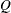is the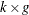side constraint coefficient matrix for nonarc variables, where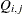is the coefficient of nonarcin theth side constraint

•is the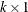side constraint right-hand-side vector

•is thearc lower flow bound vector

•is thearc capacity vector

•is thenonarc variable lower bound vector

•is thenonarc variable upper bound vector